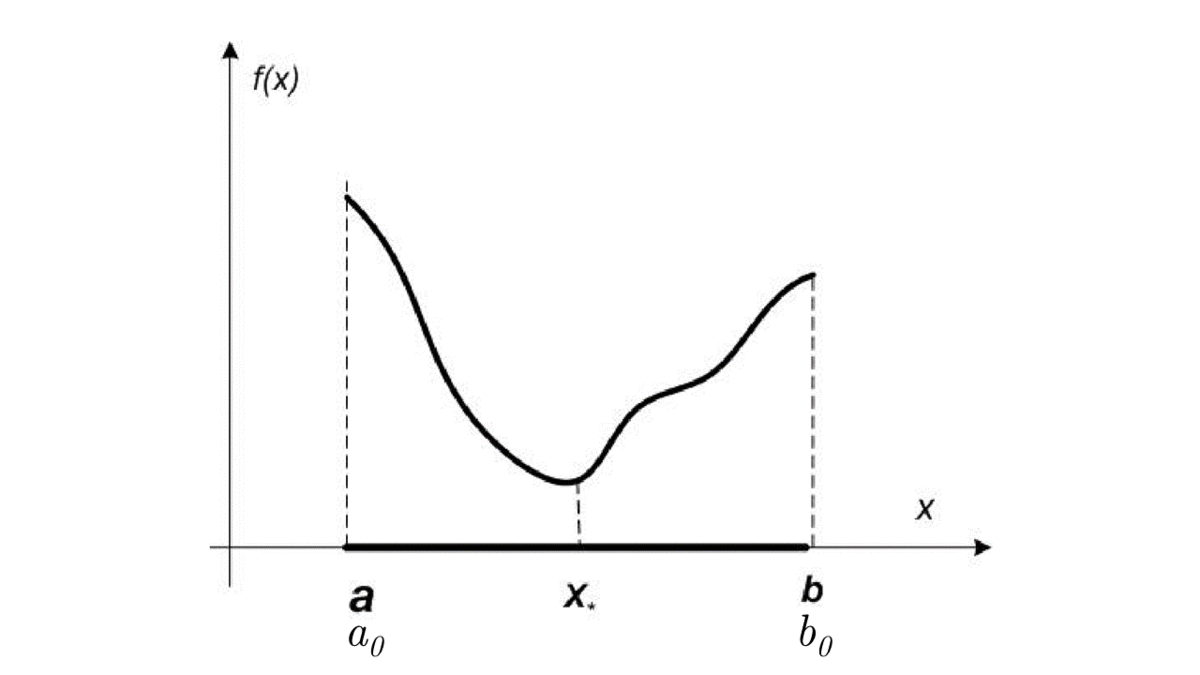# Idea

We divide a segment into two equal parts and choose the one that contains the solution of the problem using the values of functions.

# Algorithm

def binary_search(f, a, b, epsilon):
c = (a + b) / 2
while abs(b - a) > epsilon:
y = (a + c) / 2.0
if f(y) <= f(c):
b = c
c = y
else:
z = (b + c) / 2.0
if f(c) <= f(z):
a = y
b = z
else:
a = c
c = z
return c# Bounds

The length of the line segment on $k+1$-th iteration:

For unimodal functions, this holds if we select the middle of a segment as an output of the iteration $x_{k+1}$:

Note, that at each iteration we ask oracle no more, than 2 times, so the number of function evaluations is $N = 2 \cdot k$, which implies:

By marking the right side of the last inequality for $\varepsilon$, we get the number of method iterations needed to achieve $\varepsilon$ accuracy: